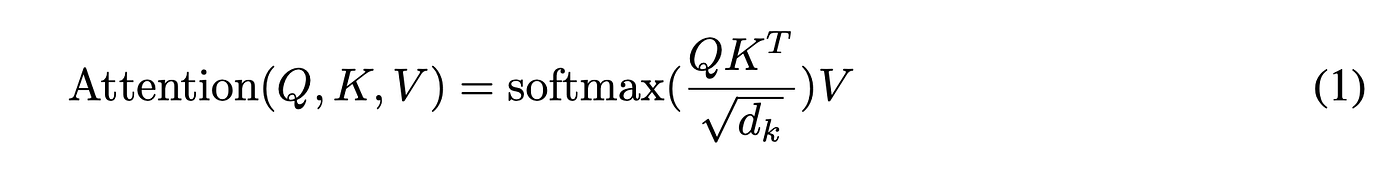# Transformer’s Scaled Dot-Product AttentionScaled Dot Product attention from 
`[10, 20, 30, …90]`
`[1, 2, 3, …9]`
`[10, 20, 30, …90]`

# Code used for the vis

`import torchfrom torch import nnfrom matplotlib import pyplot as pltf = nn.Softmax(dim=1)x = torch.tensor([[1, 2, 3, 4, 5, 6, 7, 8, 9], [0.1, 0.20, 0.3, 0.40, 0.50, 0.6, 0.7, 0.8, 0.9]], dtype=torch.float)y = f(x)plt.scatter(range(x.shape), y, c="r", label="no scale")plt.scatter(range(x.shape), y, c="g", label="scale by 10")plt.legend()plt.show()`

--

--